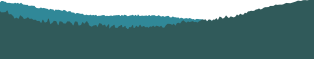PyCon 2016 in Portland, Or## Computational Statistics

Audience level:
Intermediate
Category:
Science

### Description

Statistical inference is a fundamental tool in science and engineering, but it is often poorly understood. This tutorial uses computational methods, including Monte Carlo simulation and resampling, to explore estimation, hypothesis testing and statistical modeling. Attendees will develop understanding of statistical concepts and learn to use real data to answer relevant questions.

### Abstract

Do you know the difference between standard deviation and standard error? Do you know what statistical test to use for any occasion? Do you really know what a p-value is? How about a confidence interval? Most people don’t really understand these concepts, even after taking several statistics classes. The problem is that these classes focus on mathematical methods that bury the concepts under a mountain of details. This tutorial uses Python to implement simple statistical experiments that develop deep understanding. Attendees will learn about resampling and related tools that use random simulation to perform statistical inference, including estimation and hypothesis testing. We will use pandas, which provides structures for data analysis, along with NumPy and SciPy. I will present examples using real-world data to answer relevant questions. The tutorial material is based on my book (Think Stats), a class I teach at Olin College, and my blog, “Probably Overthinking It.”

### Student Handout

No handouts have been provided yet for this tutorial